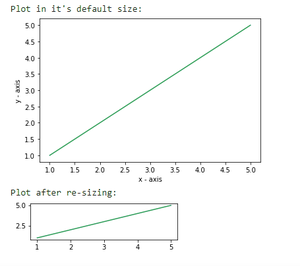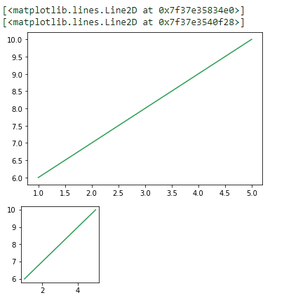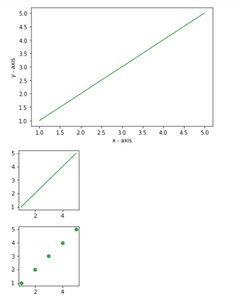# Change plot size in Matplotlib – Python

• Difficulty Level : Basic
• Last Updated : 26 Nov, 2020

Prerequisite: Matplotlib

Plots are an effective way of visually representing data and summarizing it in a beautiful manner. However, if not plotted efficiently it seems appears complicated. In python’s matplotlib provides several libraries for the purpose of data representation.

While making a plot it is important for us to optimize its size. Here are various ways to change the default plot size as per our required dimensions or resize a given plot.

Method 1: Using set_figheight() and set_figwidth()

For changing height and width of a plot set_figheight and set_figwidth are used

## Python3

 `# importing the matplotlib library ` `import` `matplotlib.pyplot as plt ` ` `  `# values on x-axis ` `x ``=` `[``1``, ``2``, ``3``, ``4``, ``5``] ` `# values on y-axis ` `y ``=` `[``1``, ``2``, ``3``, ``4``, ``5``] ` ` `  `# naming the x and y axis ` `plt.xlabel(``'x - axis'``) ` `plt.ylabel(``'y - axis'``) ` ` `  `# plotting a line plot with it's default size ` `print``(``"Plot in it's default size: "``) ` `plt.plot(x, y) ` `plt.show() ` ` `  `# plotting a line plot after changing it's width and height ` `f ``=` `plt.figure() ` `f.set_figwidth(``4``) ` `f.set_figheight(``1``) ` ` `  `print``(``"Plot after re-sizing: "``) ` `plt.plot(x, y) ` `plt.show() `

Output:Method 2: Using figsize

figsize() takes two parameters- width and height (in inches). By default the values for width and height are 6.4 and 4.8 respectively.

Syntax:

plt.figure(figsize=(x,y))

Where, x and y are width and height respectively in inches.

## Python3

 `import` `matplotlib.pyplot as plt ` ` `  `# values on x and y axis ` `x ``=` `[``1``, ``2``, ``3``, ``4``, ``5``] ` `y ``=` `[``6``, ``7``, ``8``, ``9``, ``10``] ` ` `  `# plot in it's default size ` `display(plt.plot(x, y)) ` ` `  `# changing the size of figure to 2X2 ` `plt.figure(figsize``=``(``2``, ``2``)) ` `display(plt.plot(x, y)) `

Output:output screenshot

Method 3: Changing the default rcParams

We can permanently change the default size of a figure as per our needs by setting the figure.figsize.

## Python3

 `# importing the matplotlib library ` `import` `matplotlib.pyplot as plt ` ` `  `# values on x-axis ` `x ``=` `[``1``, ``2``, ``3``, ``4``, ``5``] ` `# values on y-axis ` `y ``=` `[``1``, ``2``, ``3``, ``4``, ``5``] ` ` `  `# naming the x axis ` `plt.xlabel(``'x - axis'``) ` `# naming the y axis ` `plt.ylabel(``'y - axis'``) ` ` `  `# plotting a line plot with it's default size ` `plt.plot(x, y) ` `plt.show() ` ` `  `# changing the rc parameters and plotting a line plot ` `plt.rcParams[``'figure.figsize'``] ``=` `[``2``, ``2``] ` ` `  `plt.plot(x, y) ` `plt.show() ` ` `  `plt.scatter(x, y) ` `plt.show() `

Output:My Personal Notes arrow_drop_up
Recommended Articles
Page :